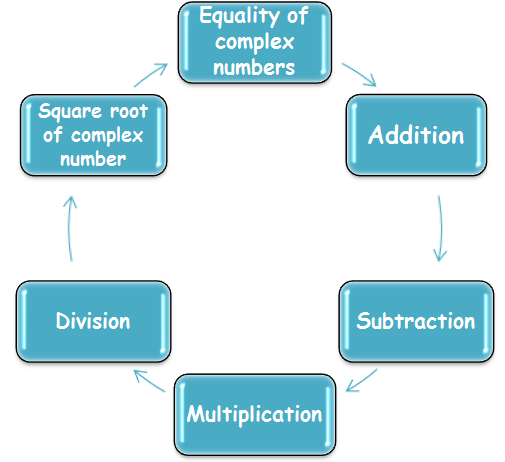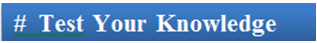×#### Thank you for registering.

One of our academic counsellors will contact you within 1 working day.

Click to Chat

1800-1023-196

+91-120-4616500

CART 0

• 0

MY CART (5)

Use Coupon: CART20 and get 20% off on all online Study Material

ITEM
DETAILS
MRP
DISCOUNT
FINAL PRICE
Total Price: Rs.

There are no items in this cart.
Continue Shopping```Algebraic Operations on Complex NumbersComplex numbers is an extremely important chapter of Algebra a sit lays the foundation fro various other chapters too. In this section, our focus would be on algebra of complex numbers which shall include equality of two complex numbers, addition, subtraction, multiplication and division of complex numbers. Finally we shall focus on computation of square root of complex number.In performing operations with complex numbers we can proceed as in the algebra of real numbers replacing i2 by -1 when it occurs.Let z1 and z2 be two complex numbers where z1 = x1 + iy1 and z2 = x2 + iy2.

Then if both the complex numbers are equal i.e. if z1 = z2 then Re(z1) = Re(z2) and Im(z1) = Im(z2).

i.e. if x1 + iy1 = x2 + iy2

Then x1 = x2 and y1 = y2 simultaneously.Let z1 and z2 be two complex numbers, z1 = x1 + iy1 and z2 = x2 + iy2.

Then the addition of the complex numbers z1 and z2  is defined as

z1 + z2 = (x1 + iy1) + ( x2 + iy2) = (x1 + x2) + i(y1 + y2) ∈ C

Re (z1 + z2) = Re (z1) + Re (z2) and

Im (z1 + z2) = Im (z1) + Im (z2)Let z1 and z2 be two complex numbers, z1 = x1 + iy1 and z2 = x2 + iy2.

Then the subtraction of the complex numbers z1 and z2 is defined as

z1 - z2 = (x1 + iy1) - ( x2 + iy2) = (x1 - x2) + i(y1 - y2) ∈ C

Re (z1 - z2) = Re(z1) - Re(z2) and

Im (z1 - z2) = Im(z1) - Im(z2)

Watch the following video for more on addition and subtraction of complex numbers:Let z1 and z2 be two complex numbers, z1 = x1 + iy1 and z2 = x2 + iy2.

Then the multiplication of the complex numbers z1 and z2  is defined as

z1.z2 = (x1 + iy1).(x2 + iy2) = (x1x2 - y1y2 ) + i(x1y2 + x2y1) ∈ C

Re(z1 z2) = Re(z1) . Re(z2) - Im(z1) . Im(z2)

Im(z1 z2) = Im(z1) . Re(z2) + Im(z2) . Re(z1)

For a real number λ and a complex number z = x + iy,

λ.z = λ(x + iy) = λx + i λy

The following properties are obvious:

(a) λ (z1 + z2) = λz1 + λz2

(b) λ1 (λ2 z) = (λ1λ2)z

(c) (λ1 + λ2)z = λ1z + λ2z for all z, z1, z2 ∈ C and λ, λ1, λ2 ∈ R.Let z1 and z2 be two complex numbers, z1 = x1 + iy1 and z2 = x2 + iy2.

Then, z1 / z2 = (x1 + iy1) /(x2 + iy2)

= (x1 + iy1) /(x2 + iy2) x (x2 - iy2)/(x2 - iy2)

= (x1x2 - x1y2i + y1x2i - y1y2i2) / (x22 – y22i2)

= [(x1x2  + y1y2) + (y1x2 – x1y2)i ]/ (x22 – y22i2)

= (x1x2  + y1y2)/ (x22 + y22) + (y1x2 – x1y2)/ (x22 + y22)i

Note:

Inequalities are not defined in case of imaginary numbers. So we can’t say one complex number > another like 4 + zi < 2 + 4i is meaningless.

Example 1:  If z = x + iy, z1/3 = a – ib and x/a – y/b = k(a2 - b2), then find the value of k.

Solution: (x + iy)1/3 = a – ib

So, x + iy = (a – ib)3

= (a3 – 3ab2) + i(b3 – 3a2b)

So, x = a3 – 3ab2, y = b3 – 3a2b

This gives x/a = a2 - 3b2 and y/b = b2 – 3a2.

So, x/a – y/b = a2 – 3b2 – b2 + 3a2 = 4(a2 - b2)

so, k = 4.

Example 2: Show that the polynomial x4p + x4q+1 + x4r+2 + x4s+3 is divisible by x3 + x2 + x + 1 where p, q, r, s ∈ N.

Solution: Let f(x) = x4p + x4q+1 + x4r+2 + x4s+3

x3 + x2 + x + 1 = (x2 + 1)(x + 1) = (x + i)(x – i)(x + 1)

f(i) = i4p + i4q+1 + i4r+2 + i4s+3 = 1 + i + i2 + i3 = 1 + i – 1 – i = 0

f(-i) = (- i)4p + (-i)4q+1 + (-i)4r+2 + (-i)4s+3 = 1 + (-i) + (-i)2 + (-i)3 = 1 – i – 1 + i = 0

f(-1) = (- 1)4p + (-1)4q+1 + (-1)4r+2 + (-1)4s+3 = 0

Thus, by division theorem f(x) is divisible by x3 + x2 + x + 1.

Example 3: If the expression (1 + ir)3 is of the form s(1 + i) for some real ‘s’ where ‘r’ is also real and i = √-1, then the value of ‘r’ can be

(a) cot π/8

(b) sec π

(c) tan π/12

(d) tan 5π/12

Solution: We have (1 + ir)3 = s(1 + i)

1+3ri + 3r2i2 + r3i3 = s(1 + i)

1-3r2 + i(3r – r3) = s + si

So, 1 – 3r2 = s = 3r – r3

Hence, 1 – 3r2 = 3r – r3

r3 – 3r2 – 3r + 1 = 0 gives (r3 + 1) – 3r(r + 1) = 0

We have (r + 1)(r2 + 1 – r – 3r) = 0

Therefore, r = -1 or r2 – 4r + 1 = 0

So, r = [4 ± √16 – 4] / 2 = [4 ± 2√3] / 2

So, r = 2 + √3 or 2 - √3.

Hence, options (b), (c) and (d) are correct.Q1. √a√b = √ab only if

(a) at least one of a or b is non-negative

(b) both a and b are non-negative

(c) at least one of a or b is positive

(d) none of these

Q2. z1/z2 =

(a) Re(z1) + Re(z2) + i {Im(z1) + Im (z2)}

(b) Re(z1/z2) + i Im(z1/z2)

(c) Re (z1) Im (z2) + i Im(z1) Re(z2)

(d) none of these

Q3. Re(z1z2) =

(a) Re(z1) + Re(z2) + i {Im(z1) + Im (z2)}

(b) Re(z1/z2) + i Im(z1/z2)

(c) Re (z1) .Re (z2) - Im(z1) Im(z2)

(d) none of these

Q4. In context of complex numbers

(a) we can talk of only equality of complex numbers

(b) there is no such concept as equality of complex numbers

(c) we can talk of inequality of complex numbers

(d) we can talk of both equality and inequality of two complex numbers

Q5. The real values of x and y for which the equation (x4 + 2xi ) – (3x2 + yi) = ( 3 – 5i) + (1 + 2yi) is satisfied.

(a) x = 2 and y = 3.

(b) x = – 2 and y = 1/3.

(c ) x = ± 2 and y = ± 3.

(d) x = ± 2, y = 3, 1/3Q1.
Q2.
Q3.
Q4.
Q5.

(a)
(b)
(c)
(a)
(d)

To read more, Buy study materials of Complex Numbers comprising study notes, revision notes, video lectures, previous year solved questions etc. Also browse for more study materials on Mathematics here.
```### Course Features

• 731 Video Lectures
• Revision Notes
• Previous Year Papers
• Mind Map
• Study Planner
• NCERT Solutions
• Discussion Forum
• Test paper with Video Solution